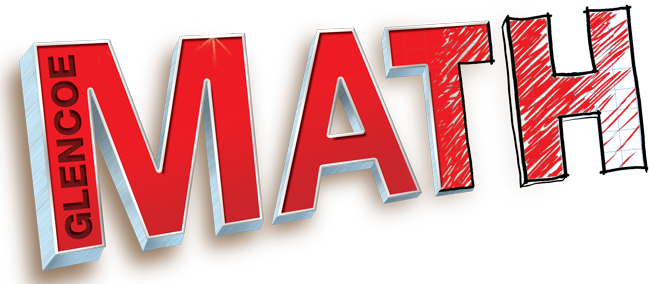•  Math Curriculum

##McGraw-Hill My Math is the program adopted for math instruction in grades 1- 5 to align with the Arizona College and Career Readiness Standards. My Math utilizes rigor, student engagement, and personalization to develop students’ understanding of math concepts. Specifically, My Math utilizes three components of rigor for math instruction: conceptual understanding, procedural skill and fluency, and application. Mathematical practices are taught in every lesson. Students use a wide-range of resources to interact with math in a meaningful way.

Parents and students can access My Math materials on the Student Portal. These materials include additional practice, the digital student book, virtual manipulatives, personal tutors, lesson animations with modeled examples and eHelp.

Glencoe MathStudents in 6th grade work with the Glencoe Math Course I text to assist in developing full and rich math abilities as a preparation for junior high math. Rigor is built-in and supported throughout the program. The three components of rigor — conceptual understanding, application, and procedural fluency — are embedded in resources, lessons, and practice state assessments.

Glencoe Math meets students wherever they are in their learning. Assessments help you determine proficiency before, during, and after lessons. Differentiated instruction resources ensure approaching-level students master concepts before moving on, while beyond-level students are continually challenged. Glencoe Math supports higher-order thinking skills, real-world applications of math, problem solving, and a deeper understanding of mathematical concepts. Glencoe engages every student to help them develop necessary critical thinking skills.

The goal of Glencoe Math is to make math relevant and rigorous, so all students can achieve math proficiency. Glencoe Math is structured to help students grasp an entire concept and understand each step of it before moving on to the next one. Students are challenged with numerous practice problems and problems with real-world application. Each lesson includes higher-order thinking problems and spiral review.

Parents and students can access Glencoe Math materials on the Student Portal. These materials include additional practice, the digital student book, virtual manipulatives, personal tutors, lesson animations with modeled examples and eHelp.

CUSD’s Math Curriculum Information